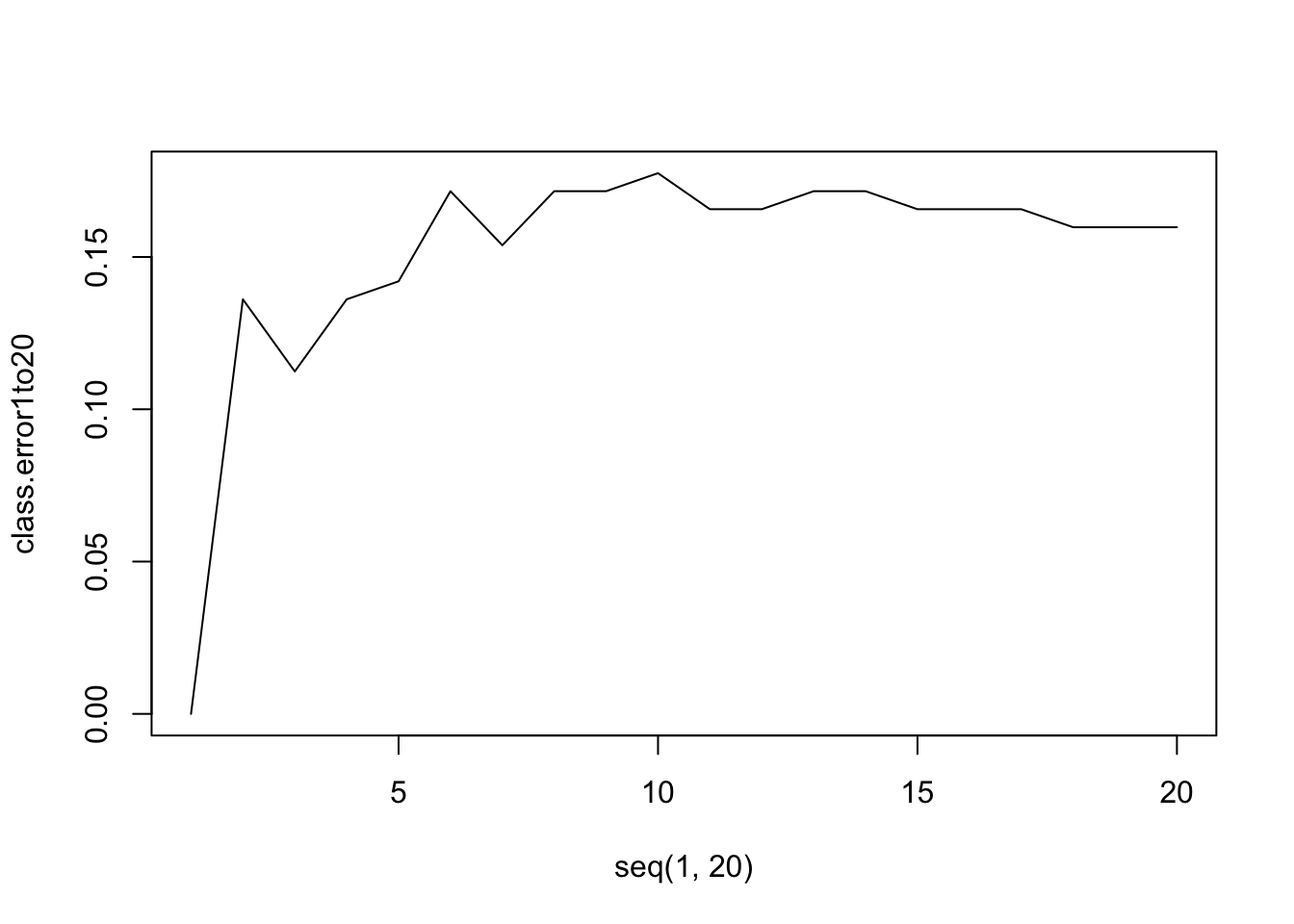# 5 K-nearest Neighbours Classification

## 5.1 Introduction

The K-nearest Neighbours (KNN) for classification, uses a similar idea to the KNN regression. For KNN, a unit will be classified as the majority of its neighbours.

Read the following chapters of An introduction to statistical learning:

## 5.3 Practical session

With the bmd.csv dataset, we will use KNN (k=3) with the variables AGE, SEX, BMI and BMD to classify FRACTURE and compute the confusion matrix

First, let’s import the dataset

#libraries that we will need
library(class)   #knn
set.seed(1974) #fix the random generator seed

bmd.data     <-
stringsAsFactors = TRUE)

bmd.data$bmi <- bmd.data$weight_kg / (bmd.data$height_cm/100)^2 let’s standardise all the variables so they have mean 0 and SD=1 so that the distances are in the same scale.  bmd.data$age.std     <- scale(bmd.data$age) bmd.data$sex.num.std <- scale(as.numeric(bmd.data$sex)) #1-F, #2-M bmd.data$bmi.std     <- scale(bmd.data$bmi) bmd.data$bmd.std     <- scale(bmd.data$bmd)  Now we use knn=3 model.knn3 <- knn(train = bmd.data[c("age.std", "sex.num.std", "bmi.std","bmd.std")], test = bmd.data[c("age.std", "sex.num.std", "bmi.std","bmd.std")], cl = bmd.data$fracture, k=3)
table(model.knn3, bmd.data$fracture) ## ## model.knn3 fracture no fracture ## fracture 38 7 ## no fracture 12 112 TRY IT YOURSELF: 1. Repeat the classification model from above but now with k=20 and compute the confusion matrix. See the solution code  model.knn20 <- knn(train = bmd.data[c("age.std", "sex.num.std", "bmi.std","bmd.std")], test = bmd.data[c("age.std", "sex.num.std", "bmi.std","bmd.std")], cl = bmd.data$fracture, k=20 )
table(model.knn20, bmd.data$fracture) 1. (hard) Plot the error rate for KNN with k=1 to 20 using the variables AGE, SEX, BMI and BMD to classify FRACTURE See the solution code knn.fit <- function(k.par){ knn.model <- knn(train = bmd.data[c("age.std", "sex.num.std", "bmi.std","bmd.std")], test = bmd.data[c("age.std", "sex.num.std", "bmi.std","bmd.std")], cl = bmd.data$fracture, k=k.par )
class.error<- 1-sum(diag(table( knn.model, bmd.data\$fracture))/169)
return(class.error)
}

class.error1to20 <- sapply(seq(1,20), knn.fit)
plot(seq(1,20), class.error1to20, type="l")## 5.4 Exercises

1. The dataset bdiag.csv, included several imaging details from patients that had a biopsy to test for breast cancer.
The variable diagnosis classifies the biopsied tissue as M = malignant or B = benign.

1. Use a KNN with k=5 to predict Diagnosis using texture_mean and radius_mean.

2. Build the confusion matrix for the classification above

3. Plot the scatter plot for texture_mean and radius_mean and draw the border line for the prediction of Diagnosis based on the model in a)

4. Plot the scatter plot for texture_mean and radius_mean and draw the border line for the prediction of Diagnosis based knn, k=15